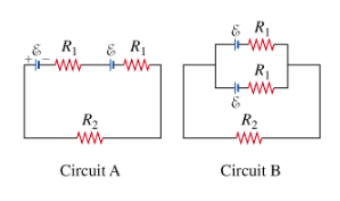# Problem: You are given two circuits with two batteries of emf ε and internal resistance R1 each. Circuit A has the batteries connected in series with a resistor of resistance R2, and circuit B has the batteries connected in parallel to n equivalent resistor.Part A. What is the current through the resistor of resistance R2 in circuit A?Part B. Calculate the current IB through the resistor of resistance R2 for circuit B?Part C. What is the power dissipated by the resistor resistance R2 for circuit A, given the ε = 10 V, R1 = 300 ohms, and R2 = 5000 ohms?Part D. For what ratio of R1 and R2 would power dissipated by the resistor of resistance R2 be the same for circuit A and circuit B?Part E. Under which of the following conditions would power dissipated by the resistance R2 in circuit A be bigger than that of circuit B?

###### FREE Expert Solution

Equivalent resistance for resistors in series:

$\overline{){{\mathbf{R}}}_{{\mathbf{eq}}}{\mathbf{=}}{{\mathbf{R}}}_{{\mathbf{1}}}{\mathbf{+}}{{\mathbf{R}}}_{{\mathbf{2}}}{\mathbf{+}}{\mathbf{.}}{\mathbf{.}}{\mathbf{.}}{\mathbf{+}}{{\mathbf{R}}}_{{\mathbf{n}}}}$

Equivalent resistance for 2 resistors in parallel:

$\overline{){{\mathbf{R}}}_{{\mathbf{eq}}}{\mathbf{=}}\frac{{\mathbf{R}}_{\mathbf{1}}{\mathbf{R}}_{\mathbf{2}}}{{\mathbf{R}}_{\mathbf{1}}\mathbf{+}{\mathbf{R}}_{\mathbf{2}}}}$

Current:

$\overline{){\mathbf{i}}{\mathbf{=}}\frac{\mathbf{V}}{\mathbf{R}}}$

Power:

$\overline{){\mathbf{P}}{\mathbf{=}}{{\mathbf{i}}}^{{\mathbf{2}}}{\mathbf{R}}}$

Part A.

Equivalent resistance, Req = R1 + R2 + R1

Req = 2R1 + R2

Total voltage:

V = ε + ε =

Since the connection is in series, the current through all the components of circuit A is the same.

89% (139 ratings)###### Problem Details

You are given two circuits with two batteries of emf ε and internal resistance R1 each. Circuit A has the batteries connected in series with a resistor of resistance R2, and circuit B has the batteries connected in parallel to n equivalent resistor.Part A. What is the current through the resistor of resistance R2 in circuit A?
Part B. Calculate the current IB through the resistor of resistance R2 for circuit B?
Part C. What is the power dissipated by the resistor resistance R2 for circuit A, given the ε = 10 V, R1 = 300 ohms, and R2 = 5000 ohms?
Part D. For what ratio of R1 and R2 would power dissipated by the resistor of resistance R2 be the same for circuit A and circuit B?
Part E. Under which of the following conditions would power dissipated by the resistance R2 in circuit A be bigger than that of circuit B?